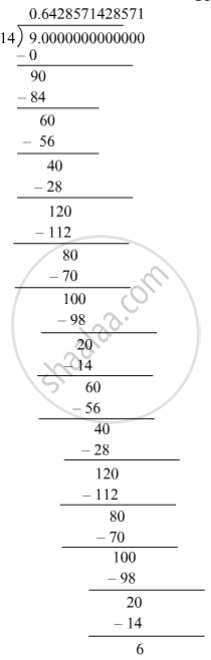SSC (English Medium) Class 8Maharashtra State Board
Share

# Write the Following Rational Number in Decimal Form. 9 14 - SSC (English Medium) Class 8 - Mathematics

ConceptConcept for Decimal Representation of Rational Numbers

#### Question

Write the following rational number in decimal form.

9/14

#### Solution

The given number is 9/4 .therefore 9/14 = 0.6428571428571.... = 0.bar6428571

"The decimal form of"  9/14  "is"  0.bar6428571.

Is there an error in this question or solution?

#### APPEARS IN

Balbharati Solution for Balbharati Class 8 Mathematics (2019 to Current)
Chapter 1: Rational and Irrational numbers
Practice Set 1.3 | Q: 3 | Page no. 4
Solution Write the Following Rational Number in Decimal Form. 9 14 Concept: Concept for Decimal Representation of Rational Numbers.
S